#### You may also likeMake a set of numbers that use all the digits from 1 to 9, once and once only. Add them up. The result is divisible by 9. Add each of the digits in the new number. What is their sum? Now try some other possibilities for yourself!### N000ughty Thoughts

How many noughts are at the end of these giant numbers?### DOTS Division

Take any pair of two digit numbers x=ab and y=cd where, without loss of generality, ab > cd . Form two 4 digit numbers r=abcd and s=cdab and calculate: {r^2 - s^2} /{x^2 - y^2}.

# Robotic Rotations

##### Age 11 to 16Challenge Level

Here is an interactivity that allows you to create rotation patterns.
Move the blue dots to create a shape and use the slider to choose an angle of rotation.

Once you've explored the interactivity, take a look at the patterns below and see if you can recreate them. If you like, you can print out the patterns.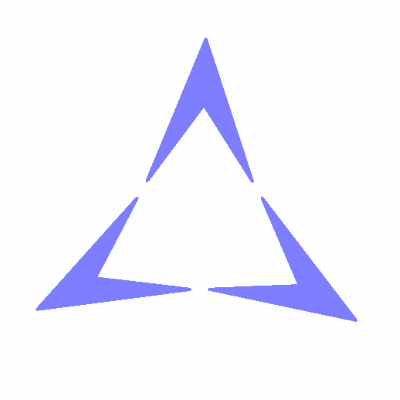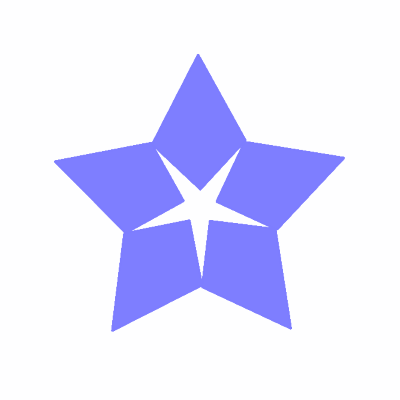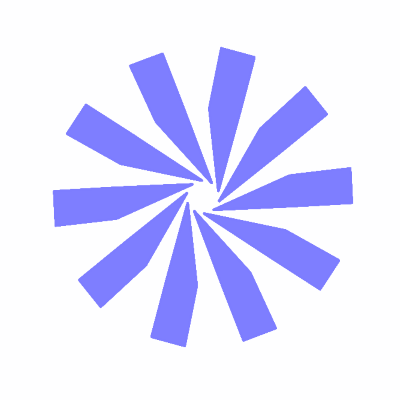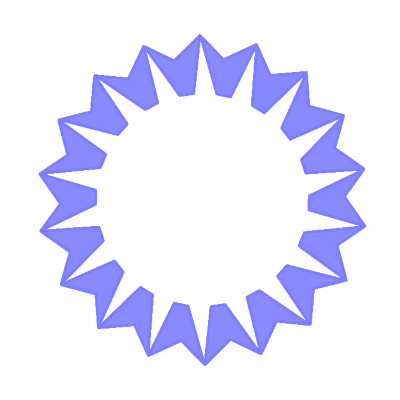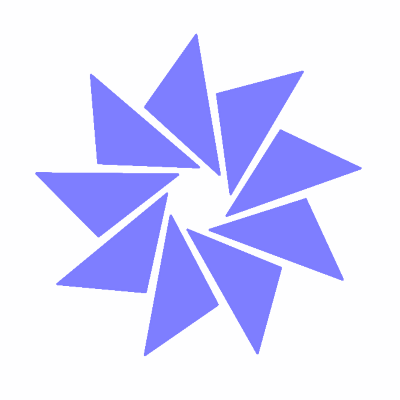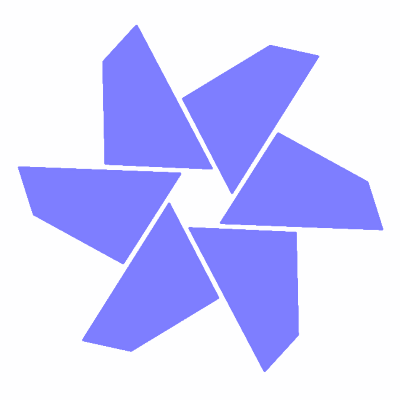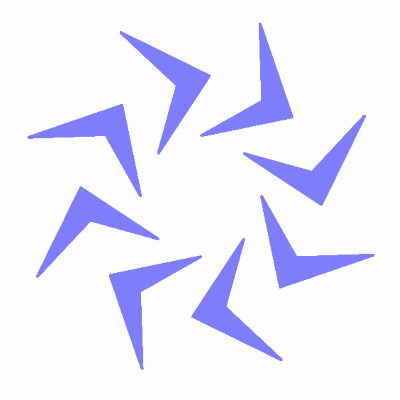How did you work out which angle to rotate by each time?To make this picture, Charlie drew a kite and then told the robot to rotate it through an angle of $144^{\circ}$.
Alison used an angle of $216^{\circ}$ and got exactly the same pattern!

What other angles could they have used?
Can the other patterns be made using more than one angle?

What is the link between the angle and the number of copies of the image in the pattern?